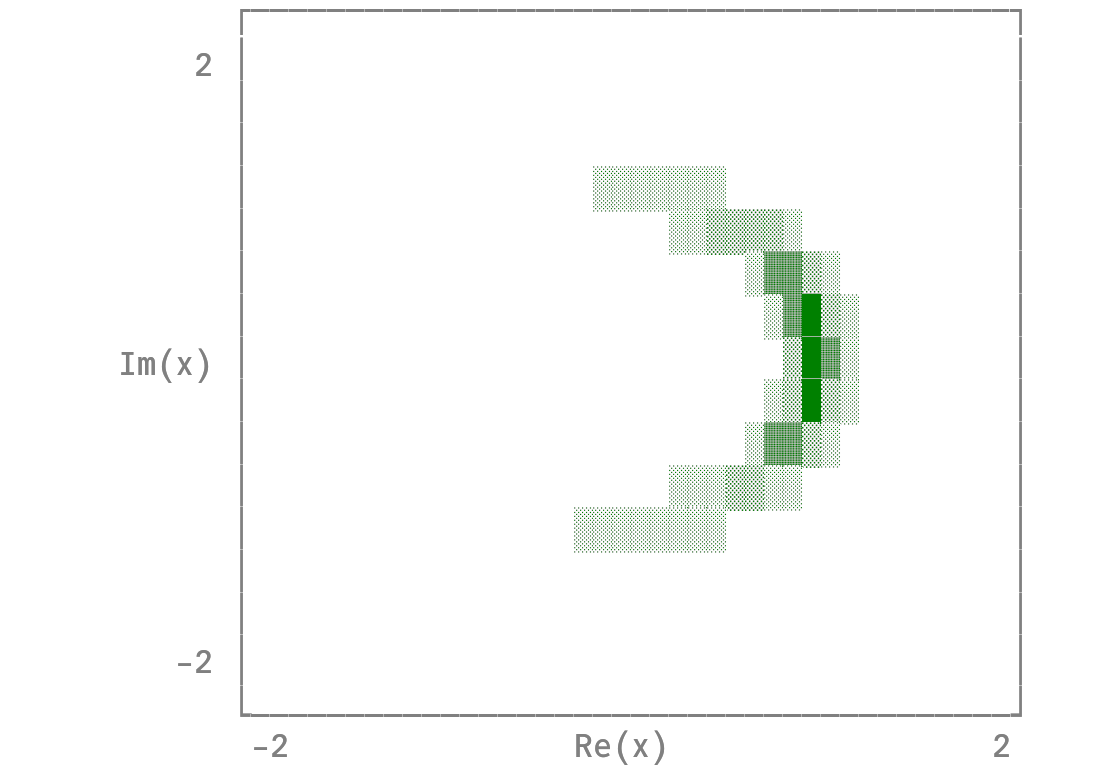# Histogram2D (complex values)using Plots
unicodeplots()
n = 10000
x = exp.(0.1 * randn(n) .+ randn(n) .* im)
histogram2d(x, nbins = (20, 40), show_empty_bins = true, normed = true, aspect_ratio = 1)